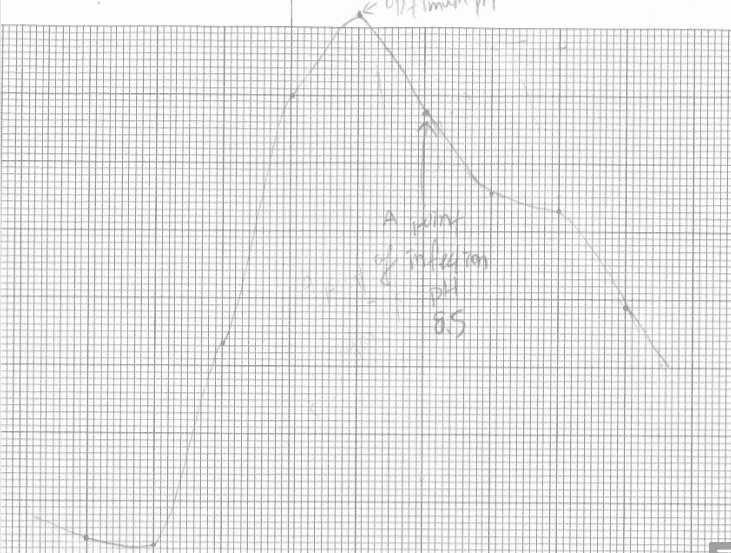# Importance of pKa to activity

• Chemistry
mimi88
Homework Statement:
Explain why the pka important to activity can be determined from the point of inflection of the graph
Relevant Equations:
ph=pka
My explanation is : for ph=pka , the number of two ionic forms[A-] and [HA] are equal,
then, for ph>pka,[A-]>[HA], the gratient gets smaller when ph is increased,
and also, for ph<pka, [A-]<[HA], the slope is becoming larger when pH is increasing .

Would u like to have a look of my explanation please.

Mentor
Point of inflection of what graph?

mimi88
a ph vs enzyme activity graph, thanks

Homework Helper
Gold Member
In agreement with Borek, the question is almost meaningless if you don't show the graph.

However I can psych that your curve looks similar to a titration curve.

Not all pH vs enzyme activity graphs look like a titration curve. They can look like two titration curves, back to back, or frequently a "bell shaped curve".

You asked us to have a look at your explanation. Assuming it looks like a titration curve I would say your explanation is not an explanation, it is a restatement.

Not just enzyme activity, but plots of many parameters that vary according to pH, for example optical absorbance (extinction coefficient) at constant wavelength, plot like a titration curve. Can you say why?

An ordinary titration curve has this same characteristic that the slope is maximum at the halfway point where pH = pK. So the main thing is if you can explain that. I don't think you can do it without bit of mathematical formulation.

mimi88As my graph has shown, my explanation is : the point of inflection is at the half of the maximum slope, where pH=pKa?

Homework Helper
Gold Member
Can you explain where this question comes from? Are these your own experiments?
The diagram is lacking even a horizontal pH scale. One would need that to even speculate.
We would need more detail but I doubt somehow that your data is accurate enough to be able to call that point a point of inflection.
The curve is not symmetrical, and I think that alone implies you'd need a model with at least three ionising groups. I doubt your data is good or extensive enough to get far with analysing that.
It would also be helpful if we knew what the system, the reaction, the measurement method is.